Question

# Flag question The outer sphere of two concentric conducting spheres of radii 9 cm and 15...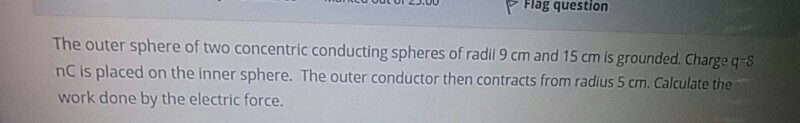Flag question The outer sphere of two concentric conducting spheres of radii 9 cm and 15 cm is grounded. Charge q=8 nC is placed on the inner sphere. The outer conductor then contracts from radius 5 cm. Calculate the work done by the electric force.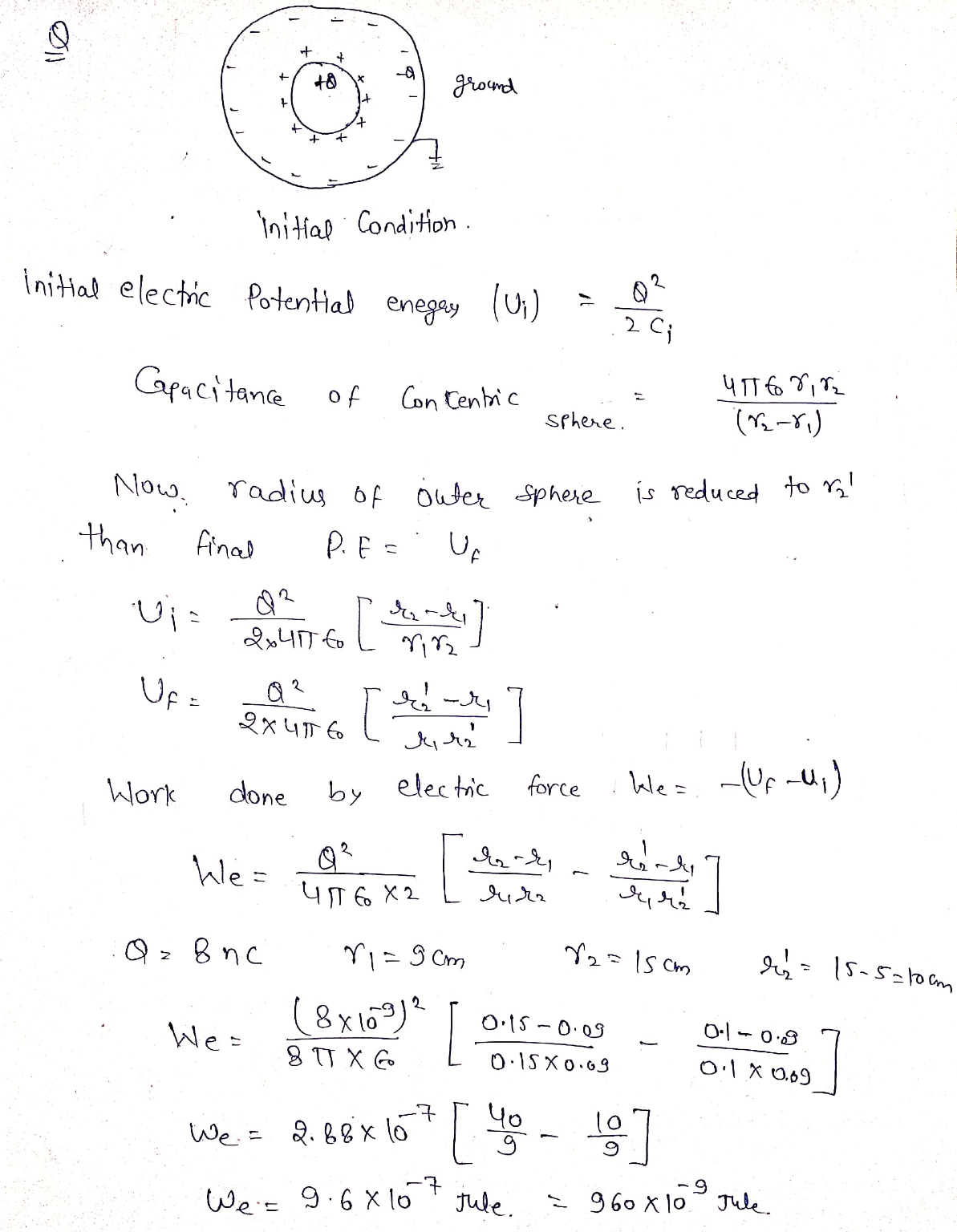#### Earn Coins

Coins can be redeemed for fabulous gifts.

Similar Homework Help Questions
• ### Consider two concentric conducting spheres. The outer sphere is a hollow shell, with an outer radius...

Consider two concentric conducting spheres. The outer sphere is a hollow shell, with an outer radius of a1 = 11.0 cm and a thickness of 0.50 cm. It initially has a charge Q1= -11.0 nC deposited on it. The inner sphere is solid of radius a2 = 5.00 cm, and it has a charge Q2 = +3.00 nC on it. (a) How much charge is on the outer surface of the shell? (b) How much charge is on the inner...

• ### Q#2: Consider two concentric conducting spheres of finite thickness in vacuum. The inner sphere has radii a, a, and car...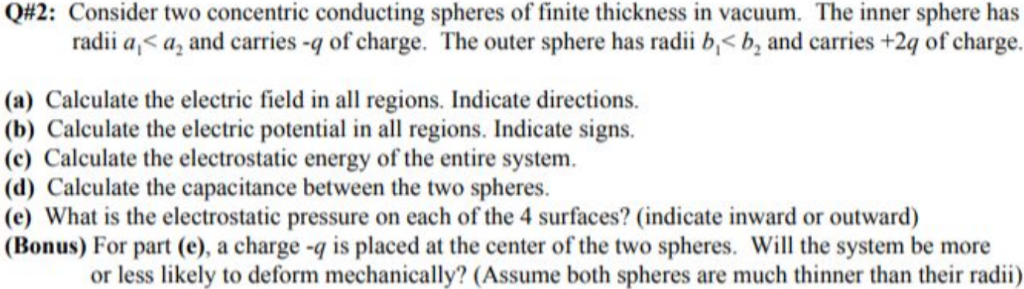Q#2: Consider two concentric conducting spheres of finite thickness in vacuum. The inner sphere has radii a, a, and carries -q of charge. The outer sphere has radii b,< b, and carries +2q of charge. (a) Calculate the electric field in all regions. Indicate directions (b) Calculate the electric potential in all regions. Indicate signs. (c) Calculate the electrostatic energy of the entire system (d) Calculate the capacitance between the two spheres. (e) What is the electrostatic pressure on each...

• ### Use Gauss’ Law to solve for the electric field everywhere. Two conducting spheres concentric spheres of...

Use Gauss’ Law to solve for the electric field everywhere. Two conducting spheres concentric spheres of different radii (e.g. r1 and r2). The inner sphere has charge –Q and the outer charge has charge +2Q.

• ### Consider two thin, concentric conducting spherical shells with radii r1 = 0.50 m and r2 =...

Consider two thin, concentric conducting spherical shells with radii r1 = 0.50 m and r2 = 1.0 m. A charge of +1.0 mC is placed on the inner sphere and a charge of +2.0 mC is placed on the outer sphere. Set the potential at infinity to be 0. Determine (a) the field inside the inner sphere, (b) the charge on the inner surface of the outer conductor, (c) the magnitude of the E-field midway between the inner and outer...

• ### Two concentric spheres have radii of 20 and 50 cm. The inner sphere has a charge...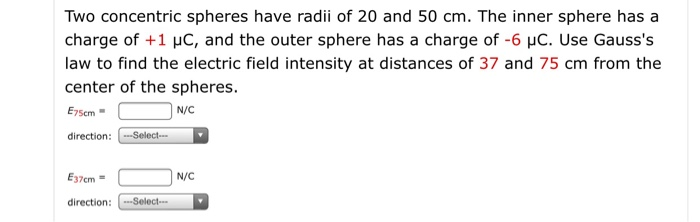Two concentric spheres have radii of 20 and 50 cm. The inner sphere has a charge of +1 pc, and the outer sphere has a charge of -6 UC. Use Gauss's law to find the electric field intensity at distances of 37 and 75 cm from the center of the spheres. E75cm - N/C direction: ---Select- E37cm - N/C direction: Select

• ### Two concentric spheres are shown in the figure. The inner sphere is a solid nonconductor and...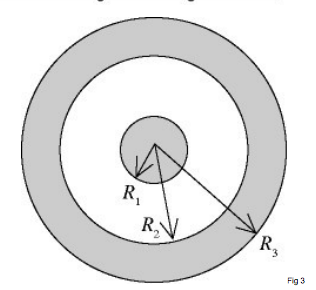Two concentric spheres are shown in the figure. The inner sphere is a solid nonconductor and carries a charge of -5.00 µC uniformly distributed over its outer surface. The outer sphere is a conducting shell that carries a net charge of 8.00 µC. No other charges are present. The radii shown in the figure have the values R1 = 10.0 cm, R2 = 20.0 cm, and R3 = 30.0 cm. (a) Find the total excess charge on the inner and...

• ### Two conducting concentric spheres (shells)have radii R1 = 10 cm and R2 = 20 cm. Both...

Two conducting concentric spheres (shells)have radii R1 = 10 cm and R2 = 20 cm. Both spheres are charged to Q = +20 C. Find: (i) The electric field within the spheres and out of the external sphere. (ii) The potential difference between the spheres. (iii) The electric field profile if the spheres are connected by a thin conducting wire.

• ### Two concentric conducting spheres of radii a = 5 cm and b = 10 cm have a potential difference V...

Two concentric conducting spheres of radii a = 5 cm and b = 10 cm have a potential difference V = 100 V between them. What is the charge carried by each sphere?Answer:a)10 nCb)100 nCc)1.1 nCd)5.5 nCe)2.2 nC

• ### Two concentric conducting spheres of radii a = 5 cm and b = 10 cm have...

Two concentric conducting spheres of radii a = 5 cm and b = 10 cm have a potential difference V = 100 V between them. What is the charge carried by each sphere?

• ### A capacitor is constructed using concentric conducting spheres. The inner sphere has radius a, the outer...

A capacitor is constructed using concentric conducting spheres. The inner sphere has radius a, the outer sphere (a thin-walled shell) has radius b. 1. Show that the capacitance is ab/k(b − a) when the space between the inner and outer spheres is empty. Start with the result from Gauss’s law for the field outside a uniform spherical charge distribution (derive the potenital difference between the spheres). 2. What is the capacitance if a = 0.1 m and b = 0.103...